• 一、matplotlib绘图样式（style） 在matplotlib中，要想设置绘制样式，最简单的方法是在绘制元素时单独设置样式。如果想保持整体风格的统一而不用对每张图一张张修改，matplotlib库还提供了四种批量修改全局样式...
一、matplotlib的绘图样式（style）
在matplotlib中，要想设置绘制样式，最简单的方法是在绘制元素时单独设置样式。如果想保持整体风格的统一而不用对每张图一张张修改，matplotlib库还提供了四种批量修改全局样式的方式。
1.matplotlib预先定义样式
matplotlib贴提供了许多内置的样式供用户使用，使用方法很简单，只需在python脚本的最开始输入想使用style的名称即可调用，尝试调用不同内置样式。
2.用户自定义stylesheet
在任意路径下创建一个后缀名为mplstyle的样式清单，编辑文件添加以下样式内容。值得特别注意的是，matplotlib支持混合样式的引用，只需在引用时输入一个样式列表，若是几个样式中涉及到同一个参数，右边的样式表会覆盖左边的值。
3.设置rcparams
通过修改默认rc设置的方式改变样式，所有rc设置都保存在一个叫做 matplotlib.rcParams的变量中。修改过后再绘图，可以看到绘图样式发生了变化。另外matplotlib也还提供了了一种更便捷的修改样式方式，可以一次性修改多个样式。
4.修改matplotlibrc文件
由于matplotlib是使用matplotlibrc文件来控制样式的，也就是上一节提到的rc setting，所以还可以通过修改matplotlibrc文件的方式改变样式。

二、在matplotlib中，设置颜色有以下几种方式：
1.RGB或RGBA
2.HEX RGB 或 RGBA
3.灰度色阶
4.单字符基本颜色
5.颜色名称
6.使用colormap设置一组颜色
有些图表支持使用colormap的方式配置一组颜色，从而在可视化中通过色彩的变化表达更多信息。
在matplotlib中，colormap共有五种类型:
顺序（Sequential）。通常使用单一色调，逐渐改变亮度和颜色渐渐增加，用于表示有顺序的信息发散（Diverging）。改变两种不同颜色的亮度和饱和度，这些颜色在中间以不饱和的颜色相遇；当绘制的信息具有关键中间值（例如地形）或数据偏离零时，应使用此值。循环（Cyclic）。改变两种不同颜色的亮度，在中间和开始/结束时以不饱和的颜色相遇。用于在端点处环绕的值，例如相角，风向或一天中的时间。定性（Qualitative）。常是杂色，用来表示没有排序或关系的信息。杂色（Miscellaneous）。一些在特定场景使用的杂色组合，如彩虹，海洋，地形等。
官方文档链接：https://matplotlib.org/3.1.0/tutorials/colors/colormaps.html

展开全文• 主要介绍了Python使用matplotlib绘图无法显示中文问题的解决方法,结合具体实例形式分析了Python使用matplotlib绘图时出现中文乱码的原因与相关解决方法,需要的朋友可以参考下
• Styles are predefined sets ...Customizing Matplotlib with style sheets and rcParams describes the mechanism and usage of styles. The Style sheets reference gives an overview of the builtin styles. matpl
样式是定义图表可视化外观的配置，它由一组预置的rcParams参数构成。matplotlib预置了一系列样式风格，可直接使用。
样式使用方法
样式相关模块为style。
1. 显示本机可用样式
matplotlib.style.available返回本机可用样式的列表。 列表只读，样式更新后，需要使用matplotlib.style.reload_library()重新加载样式。
In : import matplotlib.style as style
In : style.available
Out:
['Solarize_Light2',
'_classic_test_patch',
'bmh',
'classic',
'dark_background',
'fast',
'fivethirtyeight',
'ggplot',
'grayscale',
'seaborn',
'seaborn-bright',
'seaborn-colorblind',
'seaborn-dark',
'seaborn-dark-palette',
'seaborn-darkgrid',
'seaborn-deep',
'seaborn-muted',
'seaborn-notebook',
'seaborn-paper',
'seaborn-pastel',
'seaborn-poster',
'seaborn-talk',
'seaborn-ticks',
'seaborn-white',
'seaborn-whitegrid',
'tableau-colorblind10']

2. 显示样式详细设置
matplotlib.style.library以字典的形式返回所有样式的定义，字典键为样式名称，值为定义样式的 RcParams对象。 字典对象也是只读的，更新样式后，需要使用reload_library()重新加载样式。
In : style.library['fast']
Out:
RcParams({'agg.path.chunksize': 10000,
'path.simplify': True,
'path.simplify_threshold': 1.0})

3. 重新加载样式
matplotlib.style.reload_library()重新加载样式。
4. 使用样式
matplotlib.style.use(style)将matplotlib的绘图样式设置为某种样式。 使用default样式可以将样式为恢复到默认样式。 该函数只会更新style中定义的rcParams配置，其余rcParams配置保持不变。 参数style有四种取值：
str：样式名称或者样式文件的路径/url。通过style.available查看可用的样式名称。dict：以rcParams配置项和值为键值对的字典。Path：指向样式文件的Path对象。list：样式支持组合使用，将多个样式配置配置放置在列表中，matplotlib将逐个执行列表中每个元素的配置，元素可以为str、Path或者dict，列表右边的元素会覆盖前面元素的配置。
import matplotlib.pyplot as plt
plt.bar([1,2,3],[1,2,3])
plt.show()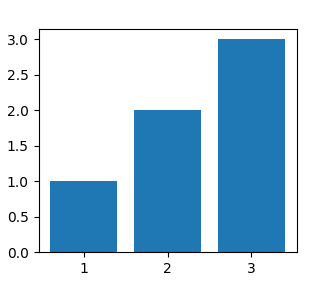import matplotlib.pyplot as plt
plt.style.use('ggplot')
plt.bar([1,2,3],[1,2,3])
plt.show()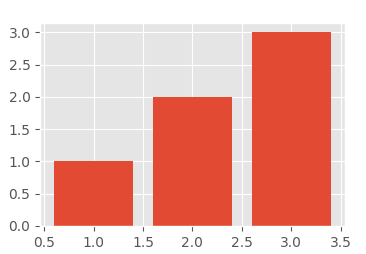import matplotlib.pyplot as plt
plt.style.use(['ggplot','dark_background'])
plt.bar([1,2,3],[1,2,3])
plt.show()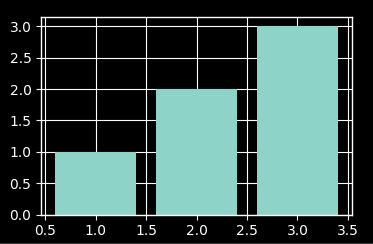import matplotlib.pyplot as plt
plt.subplot(221)
plt.bar([1,2,3],[1,2,3])
plt.style.use('ggplot')
plt.subplot(222)
plt.bar([1,2,3],[1,2,3])
plt.style.use('grayscale')
plt.subplot(223)
plt.bar([1,2,3],[1,2,3])
plt.style.use(['ggplot','grayscale'])
plt.subplot(224)
plt.bar([1,2,3],[1,2,3])
plt.show()

样式样例
参见https://matplotlib.org/gallery/style_sheets/style_sheets_reference.html
自定义样式
https://matplotlib.org/tutorials/introductory/customizing.html
展开全文• matplotlib 是python最著名的绘图库，它提供了一整套和matlab相似的命令API，十分...下面这篇文章主要给大家介绍了在python使用matplotlib绘图时图例显示问题的解决方法，需要的朋友可以参考学习，下面来一起看看吧。
•   使用matplotlib绘图的原理，主要就是理解figure(画布)、axes(坐标系)、axis(坐标轴)三者之间的关系。   以“美院学生张三写生画画”为例，来说明这三者之间的关系。   首先，张三想要画画，是不是需要在画板...
1、核心原理讲解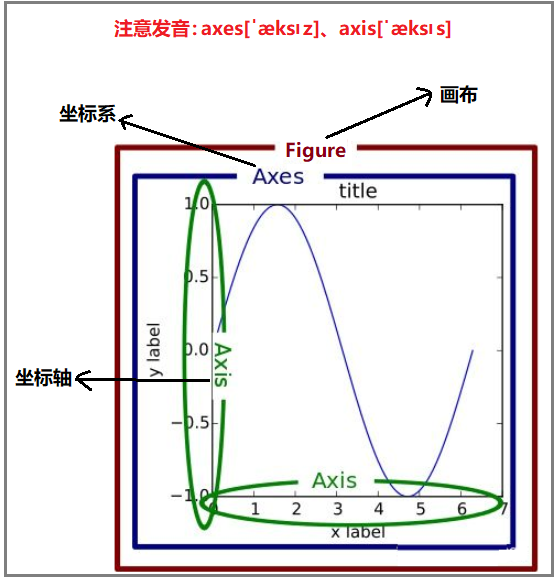使用matplotlib绘图的原理，主要就是理解figure(画布)、axes(坐标系)、axis(坐标轴)三者之间的关系。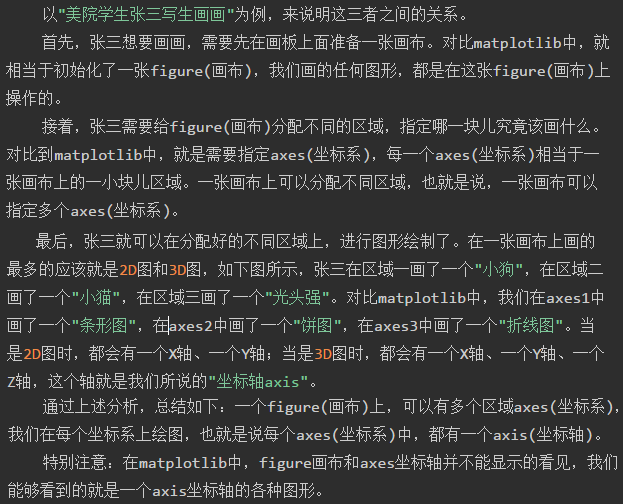张三的画板matplotlib绘图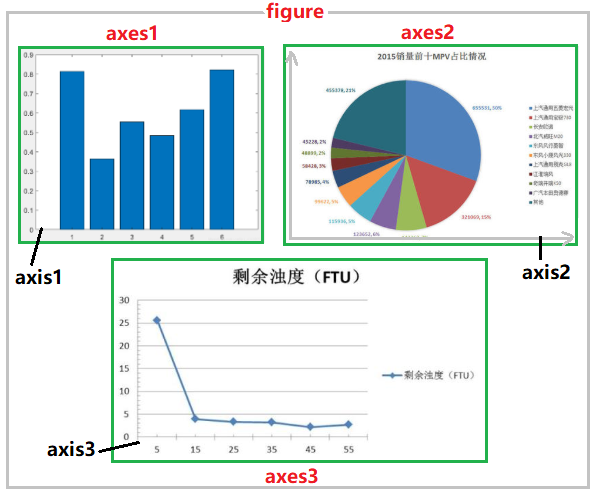2、matplotlib库的安装与导入
1）安装
pip install matplotlib

2）导入相关库
现在你不需要关注下面代码具体是什么意思，有一个主观印象即可。我将会在以后的文章中，给大家一一介绍你不懂的哪些参数。
import numpy as np
import pandas as pd
import matplotlib as mpl
import matplotlib.pyplot as plt

# 排除警告信息
import warnings
warnings.filterwarnings("ignore")

# 打印版本信息
display(np.__version__)
display(pd.__version__)
display(mpl.__version__)

# matplotlib画图常见参数设置
mpl.rcParams["font.family"] = "SimHei" # 设置字体
mpl.rcParams["axes.unicode_minus"]=False # 用来正常显示负号
plt.rcParams['font.sans-serif']=['SimHei'] # 用来正常显示中文标签

# 嵌入式显示图形
%matplotlib inline

结果如下：3、创建figure(画布)的两种方式
1）绘制一个最简单的折线图
import matplotlib as mpl
import matplotlib.pyplot as plt

x = [1,3,5,7]
y = [4,9,6,8]
plt.plot(x,y)
plt.show()

结果如下：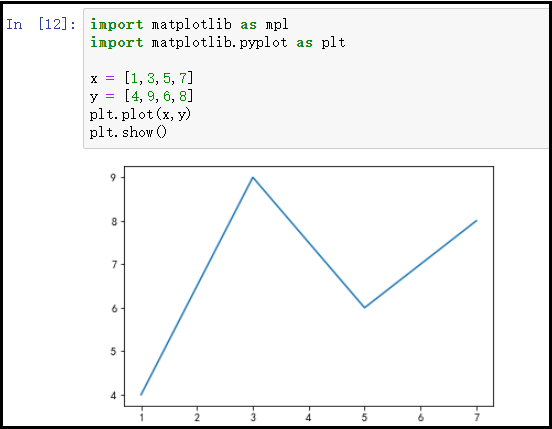结果分析：   在前面的叙述中，我们已经说过，想要使用matplotlib绘图，必须先要创建一个figure(画布)对象，然后还要有axes(坐标系)。但是观察上述代码，我们并没有创建figure对象，那么怎么又可以画图呢？   对于上述疑问，接下来我们就要讲述创建figure(画布)的两种方式。
2）创建figure(画布)的两种方式
隐式创建显示创建
① 隐式创建figure对象
当第一次执行plt.xxx()这句绘图代码时，系统会去判断是否已经有了figure对象，如果没有，系统会自动创建一个figure对象，并且在这个figure之上，自动创建一个axes坐标系(注意：默认创建一个figure对象，一个axes坐标系)。   也就是说，如果我们不设置figure对象，那么一个figure对象上，只能有一个axes坐标系，即我们只能绘制一个图形。
② 隐式创建figure对象存在的问题
优势：如果只是绘制一个小图形，那么直接使用plt.xxx()的方式，会自动帮我们创建一个figure对象和一个axes坐标系，这个图形最终就是绘制在这个axes坐标系之上的。   劣势：如果我们想要在一个figure对象上，绘制多个图形，那么我们就必须拿到每个axes对象，然后调用每个位置上的axes对象，就可以在每个对应位置的坐标系上，进行绘图，如下图所示。注意：如果figure对象是被默认创建的，那么我们根本拿不到axes对象。因此，需要我们显示创建figure对象。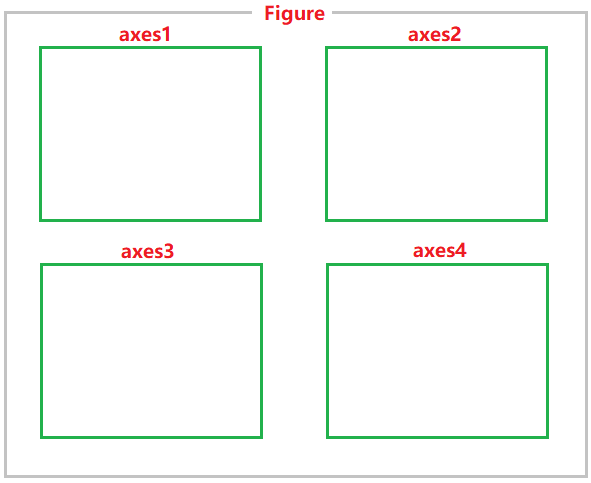③ 显示创建figure对象：以后的文章会详细讲述布局设置
# 手动创建一个figure对象
figure = plt.figure()
# 获取每个位置的axes对象

如图所示：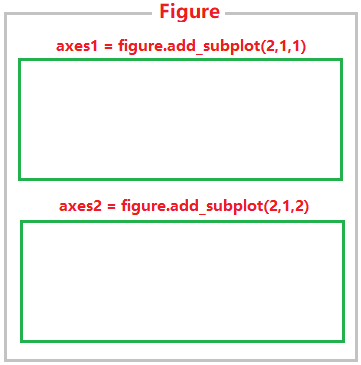举例说明：
figure = plt.figure()

axes1.plot([1,3,5,7],[4,9,6,8])
axes2.plot([1,2,4,5],[8,4,6,2])
figure.show()

结果如下：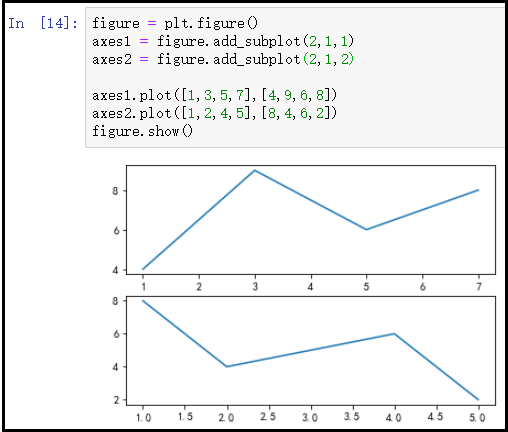4、完整的绘图步骤
① 导库
import matplotlib as mpl
import matplotlib.pyplot as plt

② 创建figure画布对象
    如果绘制一个简单的小图形，我们可以不设置figure对象，使用默认创建的figure对象，
当然我们也可以显示创建figure对象。
如果一张figure画布上，需要绘制多个图形。那么就必须显示的创建figure对象，然后
得到每个位置上的axes对象，进行对应位置上的图形绘制。

③ 根据figure对象进行布局设置
1*1
1*2
2*1
2*2
...

④ 获取对应位置的axes坐标系对象
figure = plt.figure()

⑤ 调用axes对象，进行对应位置的图形绘制
    这一步，是我们传入数据，进行绘图的一步。对于图形的一些细节设置，都可以在这一步进行。

⑥ 显示图形
    plt.show()或figure.show()
如果在pycharm中绘图的话，必须要加这句代码，才能显示。如果在notebook中进行绘图，
可以不用加这句代码，而是自动显示。

5、绘图技巧(细节设置)
* figure 画布
* axes 坐标系，一个画布上可以有多个坐标系
* axis 坐标轴，一个坐标系中可以有多个坐标轴，一般都是二维平面坐标系，或者三维立体坐标系
* title 标题
* legend 图例
* grid 背景网格
* tick 刻度
* axis label 坐标轴名称
* tick label 刻度名称
* major tick label 主刻度标签
* minor tick label 副刻度标签
* line 线
* style 线条样式
* marker 点标记
* font 字体相关

通过对matplotlib绘图的原理，有所了解之后，我们需要学习的就是常见的matplotlib绘图技巧，常见的绘图技巧如上所示，这些绘图技巧都能够很好的帮助我们画出更美观、更直观的图形。下一节，我将会讲述matplotlib绘图技巧(千万不要错过)。
展开全文matplotlib
• 目录1 marker 标号1.1 相关参数2 linestyle 线的样式3 linewidth 线宽4 label 图例5 alpha 透明度6 color 颜色创建自己的colormapmatplotlib.colors.ListedColormap( n*4维数组 或 [“b”,”y”,”g”] 形式的列表 )...
• matplotlib绘图的完善及美化 --0、导入基本库-- import numpy as npimport pandas as pdimport matplotlib as mplimport matplotlib.pyplot as plt #最常用的绘图库 mpl.rcParams["font.family"]="SimHei" #使用...
目录
matplotlib绘图的完善及美化
--0、导入基本库--
--1、完善标题--
--2、完善标签(图例标签设置)--
--3、轴标签说明设置--
--4、设置x与y轴的刻度范围--
--5、自定义每个刻度的显示标签--
--6、设置坐标轴的刻度间隔--
--7、网格设置--
--8、颜色，线型，点标记，透明度设置--
--9、添加注解--
--10、给图形上的坐标点添加注释--
--11、其他优化--
--12、图形样式的选择--
--13、绘制面板图、仪表板--
--14、案例--

matplotlib的绘图原理及流程详解，参考公众号上一文：matplotlib的绘图原理及流程详解
matplotlib绘图的完善及美化

--0、导入基本库--
import numpy as npimport pandas as pdimport matplotlib as mplimport matplotlib.pyplot as plt  #最常用的绘图库
mpl.rcParams["font.family"]="SimHei"  #使用支持的黑体中文字体mpl.rcParams["axes.unicode_minus"]=False # 用来正常显示负数  "-"plt.rcParams['font.sans-serif']=['SimHei'] # 用来正常显示中文标签# mpl.rcParams["font.style"]="normal"   #设置标签风格，normal 常规（默认）/italic 斜体/oblique 倾斜# mpl.rcParams["font.size"]=11   #font.size 字体的大小（默认10）# % matplotlib inline  #jupyter中用于直接嵌入图表，不用plt.show()import warningswarnings.filterwarnings("ignore") #用于排除警告
#用于显示使用库的版本print("numpy_" + np.__version__)print("pandas_" + pd.__version__)print("matplotlib_"+ mpl.__version__)

--1、完善标题--
在每个axes绘图后都应该添加一个title,使每个axes都有一个标题
默认添加一个title
plt.title("TitleName")
当然可以通过局部设置美化我们的标题
参数说明：
family=['SimHei','STSong','STXihei','NSimSun','STXihei',...]#字体
styles=['normal','italic','oblique']#字体的风格["常规(默认)","斜体字","倾斜自"]
weights=['light','normal','medium','semibold','bold','heavy','black']#字体粗细
color=["white","black","red","blue","yellow","green",...]#字体颜色
size=[10,12,14,15,16,18,22,24,....]#字体大小
案例：
font={"family":"SimHei","style":"normal","weight":"normal","color":"green","size":16}
plt.title("TitleName",fontdict=font)#显示一个中文标题
x = range(1,11)y = np.random.rand(10)z = np.random.rand(10)*2figure = plt.figure(num="案例1",figsize=(16,9),facecolor="red",edgecolor="blue",frameon=True)font={"family":"SimHei","style":"normal","weight":"normal","color":"green","size":16}
axes1 = plt.subplot(2,2,1)axes1.plot(x,z,label="折线图")plt.legend(loc="best")plt.title("图1",fontdict=font)
axes2 = plt.subplot(2,2,2)axes2.bar(x,y,label="柱状图")plt.legend(loc="best")plt.title("图2",fontdict=font)
axes3 = plt.subplot(2,2,3)axes3.scatter(x,y)plt.legend(range(1,11),loc="right",frameon=False,title="散点图",ncol=1)plt.title("图3",fontdict=font)
axes4 = plt.subplot(2,2,4)axes4.pie(y)plt.legend(range(1,11),loc="right",frameon=False,title="饼图",ncol=1)plt.title("图4",fontdict=font)
plt.show()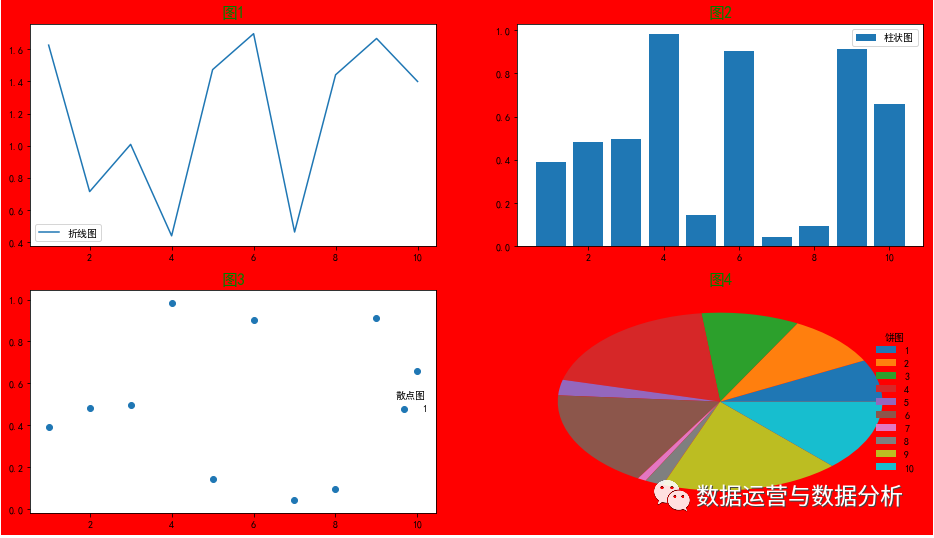--2、完善标签(图例标签设置)--
在做图的时候，尤其是一个axes中有多个图形对象的时候，必须加上标签，才能区分每个图形表示的是什么。
方法1：在绘图的时候就加上标签，label="标签名称"plt.legend(loc="best")调用生成标签
x,y=list(range(1,13)),np.random.randint(50,100,12)plt.plot(x,y,label="2016年")plt.plot(list(range(1,13)),np.random.randint(50,100,12),label="2017年")plt.legend(loc="best")#调用生成标签plt.show()
方法2：绘制完图片后，再使用plt.legend()生成标签
参数说明：
["标签名2","标签名3","标签名3",...]#一个axes中有几个表示不用意义的图就有几个标签名  loc=["best","upper","down","left","right","upperleft","upperright","downleft"...]  #loc控制图例的显示位置，默认为best，即选择一个最好的位置。  frameon=[False,True]#是否显示边框  title="标签组的大标题"#给整个标签组起一个名  ncol=n#n是数字，表示标签组显示成多少个列
案例：
x,y=list(range(1,13)),np.random.randint(50,100,12)x,y=list(range(1,13)),np.random.randint(50,100,12)plt.plot(x,y)plt.plot(list(range(1,13)),np.random.randint(50,100,12))plt.legend(["2018年","2019年"],loc="best",frameon=False,title="年降雨量",ncol=2)plt.show()

--3、轴标签说明设置--
plt.xlabel()设置x轴的标签说明。
plt.ylabel()设置y轴的标签说明。
每个axes对象都应该设置轴标签。
当然也可以通过局部设置美化我们的轴标签
案例：
x,y=list(range(1,13)),np.random.randint(50,100,12)plt.plot(x,y)plt.plot(list(range(1,13)),np.random.randint(50,100,12))plt.legend(["2018年","2019年"],loc="best",frameon=False,title="年降雨量",ncol=2)font={"family":"SimHei","style":"normal","weight":"normal","color":"green","size":12}plt.xlabel("月",fontdict=font)plt.ylabel("降雨量",fontdict=font)plt.show(

--4、设置x与y轴的刻度范围--
plt.xlim(xmin, xmax)
plt.ylim(ymin, ymax)
x,y=list(range(1,13)),np.random.randint(50,100,12)plt.plot(x,y)plt.plot(list(range(1,13)),np.random.randint(50,100,12))plt.legend(["2018年","2019年"],loc="best",frameon=False,title="年降雨量",ncol=2)font={"family":"SimHei","style":"normal","weight":"normal","color":"green","size":12}plt.xlabel("月",fontdict=font)plt.ylabel("降雨量",fontdict=font)plt.xlim(0,12)plt.ylim(50,120)plt.show()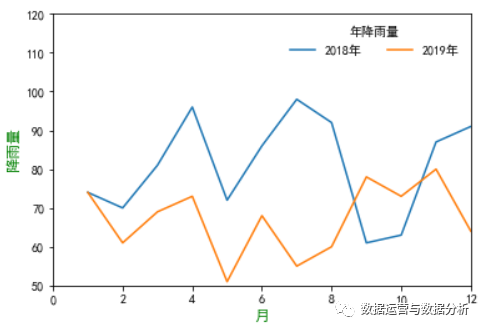--5、自定义每个刻度的显示标签--
plt.xlim([0,12])
plt.xlim(0,12)
plt.xticks([原刻度标签列表],[新的刻度标签列表])
x,y=list(range(1,13)),np.random.randint(50,100,12)plt.plot(x,y)plt.plot(list(range(1,13)),np.random.randint(50,100,12))plt.legend(["2018年","2019年"],loc="best",frameon=False,title="年降雨量",ncol=2)font={"family":"SimHei","style":"normal","weight":"normal","color":"green","size":12}plt.xlabel("月",fontdict=font)plt.ylabel("降雨量",fontdict=font)plt.xlim(0,12)plt.ylim(50,120)plt.xticks([1,2,3,4,5,6,7,8,9,10,11,12],["1月","2月","3月","4月","5月","6月","7月","8月","9月","10月","11月","12月"])plt.show(

--6、设置坐标轴的刻度间隔--
from matplotlib.pyplot import MultipleLocator
#从pyplot导入MultipleLocator类，这个类用于设置刻度间隔
x_major_locator=MultipleLocator(1)
#把x轴的刻度间隔设置为1，并存在变量里
y_major_locator=MultipleLocator(20)
#把y轴的刻度间隔设置为10，并存在变量里
ax=plt.gca()
#ax为两条坐标轴的实例
ax.xaxis.set_major_locator(x_major_locator)
#把x轴的主刻度设置为1的倍数
ax.yaxis.set_major_locator(y_major_locator)
#把y轴的主刻度设置为10的倍数
x,y=list(range(1,13)),np.random.randint(50,100,12)plt.plot(x,y)plt.plot(list(range(1,13)),np.random.randint(50,100,12))plt.legend(["2018年","2019年"],loc="best",frameon=False,title="年降雨量",ncol=2)font={"family":"SimHei","style":"normal","weight":"normal","color":"green","size":12}plt.xlabel("月",fontdict=font)plt.ylabel("降雨量",fontdict=font)plt.xlim(1,12)plt.ylim(50,110)
from matplotlib.pyplot import MultipleLocator#从pyplot导入MultipleLocator类，这个类用于设置刻度间隔x_major_locator=MultipleLocator(1)#把x轴的刻度间隔设置为1，并存在变量里y_major_locator=MultipleLocator(20)#把y轴的刻度间隔设置为10，并存在变量里ax=plt.gca()#ax为两条坐标轴的实例ax.xaxis.set_major_locator(x_major_locator)#把x轴的主刻度设置为1的倍数ax.yaxis.set_major_locator(y_major_locator)#把y轴的主刻度设置为10的倍数
plt.show()

--7、网格设置--
plt.grid()：
可以通过plt的grid方法来设置是否显示网格。
True为显示，False不显示。ax.grid(color='r',linestyle='-',linewidth=2)
color：设置网格线颜色  axis：设置网格线显示x，y或者全部显示（x，y，both）  linestyle：设置网格线形状  linewidth：设置网格线宽度
案例：
plt.grid(True,color="g",axis="both",ls="--",lw=0.5)#设置网格线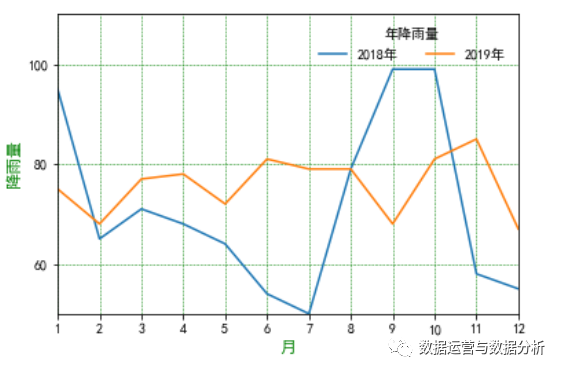--8、颜色，线型，点标记，透明度设置--
我们可以在绘制图形时，显式指定图形的颜色，点标记或线条形状。具体设置可以查看帮助文档。
color(c)：线条颜色。  linestyle(ls)：线条形状。  linewidth(lw)：线宽。  marker：点标记形状。  markersize(ms)：点标记的大小。  markeredgecolor(mec)：点边缘颜色。  markeredgewidth(mew)：点边缘宽度。  markerfacecolor(mfc)：点的颜色。  alpha：透明度（0-1之间）
说明：
颜色，点标记与线型可以使用一个组合参数进行设置。  颜色除了可以使用预设简写的字符之外，也可以使用全称（例如red）也可以使用RGB颜色表示。  颜色:[蓝色:'b'/绿色:'g'/红色:'r'/青色:'c'/品红:'m'/黄色:'y'/黑色:'k'/白色:'w']  线型:[直线:'-'/虚线:'--'/点线:':'/点划线:'-.']  点标记:[点:'.'/像素:','/圆:'o'/方形:'s'/三角形:'^']  关于这三种样式的设置有一种简写的方式：颜色，线条样式，点标记样式"颜色线条样式点标记样式"====>
案例：
x,y = list(range(1,13)),np.random.randint(50,100,12)
plt.plot(x,y,"b:o",alpha=0.5)
plt.plot(x,y,c="g",ls="--",lw=5,marker="o",ms=8,mec="r",mew=3,mfc="y",alpha=0.5)--9、添加注解--
我们可以在图形上绘制文本等说明信息（注解）。
方法1：普通文本（当前位置）--常用
plt.text()：显示文本（基于坐标）如：plt.text(6,0,"年中")
plt.figtext()：显示文本（基于图片比例定位，左下角为原点）如：plt.figtext(0.5,0.5,"图片中央")
x,y = list(range(1,13)),np.random.randint(50,100,12)
plt.plot(x,y,"b:o",alpha=0.5)plt.text(6,50,"年中")plt.figtext(0.5,0.5,"图片中央")plt.show()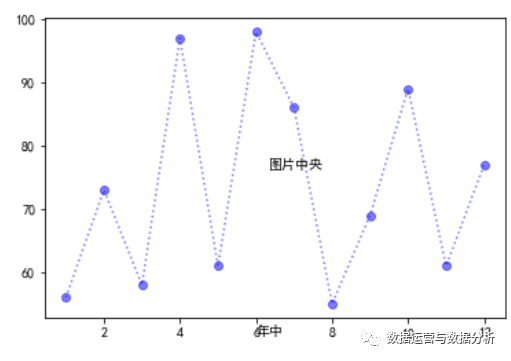方法2：连线位移（添加线条，在指定位置）--不常用
plt.arrow根据起点坐标（x，y）与各自轴的长度（x+dx,y+dy）绘制箭头。
width箭头尾部的宽度。
案例：
plt.text(4,105,"年中")
x,y = list(range(1,13)),np.random.randint(50,100,12)plt.plot(x,y,"b:o",alpha=0.5)plt.arrow(7,55,-1,-5,width=0.1,head_width=0.5,head_length=0.5,color="r")plt.text(7,55,"年中")plt.show(
方法3：箭头与文本--常用
plt.annotate():显示箭头与文本。
更多参数的使用方法自行搜索...
案例：
plt.annotate("年中",xy=(6,100),xytext=(4,105),
color="g",size=16,bbox=dict(fc="b",ec="g"),
x,y = list(range(1,13)),np.random.randint(50,100,12)plt.plot(x,y,"b:o",alpha=0.5)plt.annotate("年中",xy=(6,50),xytext=(4,80),color="g",size=16,bbox=dict(fc="b",ec="g"),arrowprops=dict(width=3,facecolor="r",headwidth=10,shrink=0.01,headlength=10))plt.show()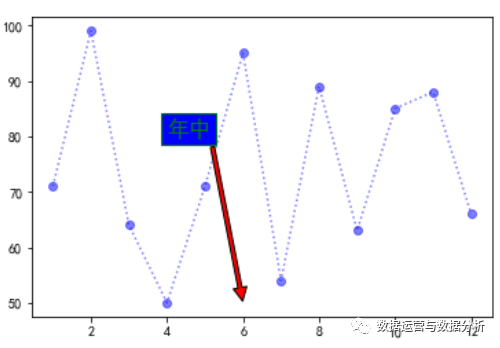--10、给图形上的坐标点添加注释--
x,y = list(range(1,13)),np.random.randint(50,100,12)plt.plot(x,y,"b:o",alpha=0.5)#添加xyfor xy in zip(x,y):    plt.annotate("(%s,%s)" % xy, xy=xy, xytext=(-5, +5), textcoords='offset points')plt.show()
x,y = list(range(1,13)),np.random.randint(50,100,12)plt.bar(x,y,alpha=0.5,color="c")#只添加yfor x, y in zip(x, y):  # zip是将X，Y1分别传到x,y中，传两个    plt.text(x, y + 0.5, '%.1f' % y, ha='center', va='bottom')  # ha,va规定坐标表示的点，默认左下plt.show()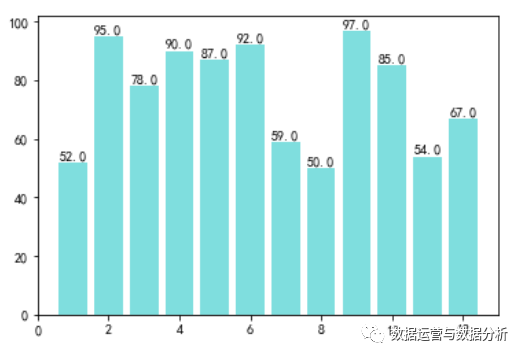--11、其他优化--
还有很多细节可以优化的，这些细节如图片的大小修改，绘图样式设置等，在作图的过程中根据实际需求查阅资料进行修改。

--12、图形样式的选择--
matplotlib可以绘制很多图形折线图、饼图、箱线图、散点图、柱状图等，更具业务需求选择不同图形对应的函数即可，
每种图形的参数都大致相同，可以查看官网图例修改即可https://matplotlib.org/gallery.html

--13、绘制面板图、仪表板--
综合型的多个图形的组合图。
自定义创建figure，自定义创建多个axes（平铺式分布的axes，内嵌式分布的axes），去参考绘图流程的第2，3条。
可视化仪表板，关注公众后续更新！！！

--14、案例--
a = range(1,11)b = np.random.rand(10)c = np.random.rand(10)*2figure = plt.figure(num="案例1",figsize=(16,9),facecolor="grey",edgecolor="blue",frameon=True)font={"family":"SimHei","style":"normal","weight":"normal","color":"black","size":16}aaxes1 = plt.subplot(2,2,1)axes1.plot(a,c,"r:o",alpha=0.5,label="折线图")plt.legend(loc="best")plt.title("图1",fontdict=font)#只添加yfor x, y in zip(a, c):  # zip是将X，Y1分别传到x,y中，传两个    axes1.text(x, y + 0.01, '%.1f' % y, ha='center', va='bottom')  # ha,va规定坐标表示的点，默认左下    axes2 = plt.subplot(2,2,2)axes2.bar(a,b,alpha=0.5,color="c",label="柱状图")plt.legend(loc="best")plt.title("图2",fontdict=font)#只添加yfor x, y in zip(a, b):  # zip是将X，Y1分别传到x,y中，传两个    axes2.text(x, y + 0.01, '%.1f' % y, ha='center', va='bottom')  # ha,va规定坐标表示的点，默认左下from matplotlib.pyplot import MultipleLocator#从pyplot导入MultipleLocator类，这个类用于设置刻度间隔x_major_locator=MultipleLocator(1)#把x轴的刻度间隔设置为1，并存在变量里y_major_locator=MultipleLocator(20)#把y轴的刻度间隔设置为10，并存在变量里ax=plt.gca()#ax为两条坐标轴的实例ax.xaxis.set_major_locator(x_major_locator)#把x轴的主刻度设置为1的倍数ax.yaxis.set_major_locator(y_major_locator)#把y轴的主刻度设置为10的倍数
axes3 = plt.subplot(2,2,3)axes3.scatter(a,b)plt.legend(range(1,11),loc="right",frameon=False,title="散点图",ncol=1)plt.title("图3",fontdict=font)plt.grid(True,color="g",axis="both",ls="--",lw=0.5)#设置网格线
axes4 = plt.subplot(2,2,4)axes4.pie(b,autopct='%1.1f%%')plt.legend(range(1,11),loc="right",frameon=False,title="饼图",ncol=1)plt.title("图4",fontdict=font)
plt.show()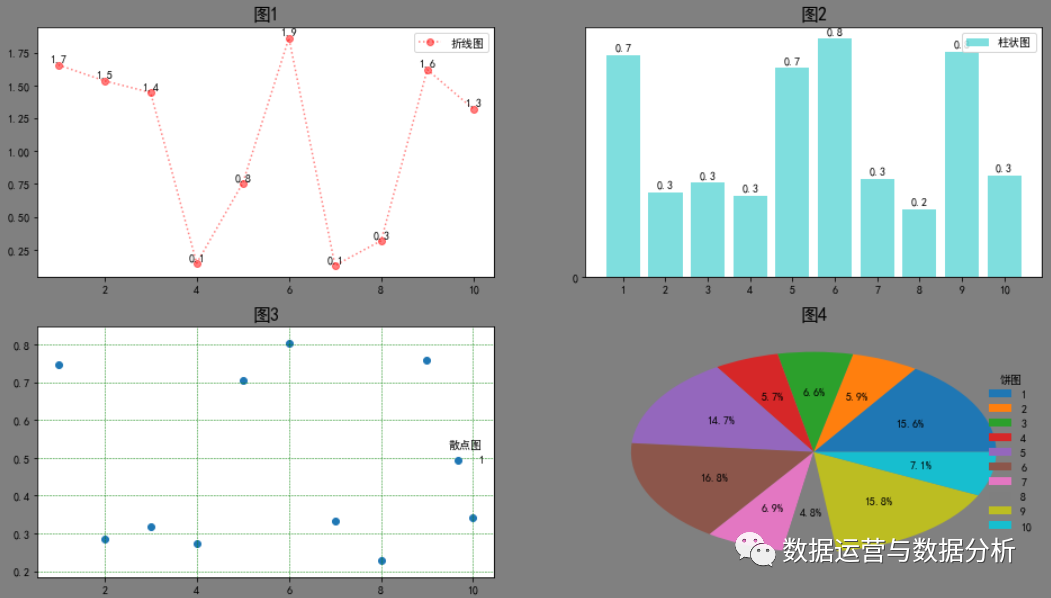展开全文python 数据可视化 数据分析 可视化
• 本文介绍如何一次性修改绘图的风格，而不用每绘制一张图都单独设置。随后介绍了绘图库中各种颜色的表达方式。此外，还大致的介绍了一些颜色的知识，最后引出了colormap的概念
• 3,4,5],[1,4,9,16,25],'-.',color = 'r') # 可以把样式和颜色写一块： plt.plot(range(100), [x**2 for x in range(100)], 'r:') linestyle属性的取值： 字符 类型 ’ - ’ 实线 ’ – ’ 虚线 ’ -. ’ 虚点线 ’ :...
• 一、matplotlib绘图样式（style） matplotlib库提供了四种批量修改全局样式的方式, 而不用对每张图一张张修改 1.matplotlib预先定义样式 matplotlib提供了许多内置的样式供用户使用，在python脚本的最开始输入...
• matplotlib绘图练习 按要求绘制：绘制一个大小为 8*4 的图；第一个图表：x 和 y，颜色是红色，线的粗细为 2（默认为 1）；标签是 sin(x)第二个图像：x 和 z 的函数图像，颜色是绿色（g 代表的绿色），线条的样式是...可视化
• matplotlib绘图工具简介 Matplotlib是一个综合库，用于在Python中创建静态，动画和交互式可视化。我们可以将通过numpy分析得到的数据结果通过matplotlib描绘出来. 配置画布 画布中图形具有的字体颜色,背景颜色等可以...数据可视化 可视化 python canvas css
• python绘图的那点小知识初次认识Matplotlib遇到问题 初次认识Matplotlib 其实对于Matplotlib我是一个相爱相杀的库，也有由于其是一个 Python 的 2D绘图库，它以各种硬拷贝格式和跨平台的交互式环境生成出版质量级别...python
• ## Matplotlib绘图秘籍

千次阅读 2020-08-01 18:50:26
Matplotlib 绘图秘籍 零、前言 一、第一步 二、自定义颜色和样式 三、处理标注 四、处理图形 五、文件输出 六、处理地图 七、处理 3D 图形 八、用户界面 贡献指南 本项目需要校对，欢迎大家提交 Pull Reques.
• Matplotlib绘图基本操作声明绘制简易折线图子图操作条形图与散点图条形图散点图直方图和盒图 声明 本文涉及的代码基于python 3.6.5 numpy1.14.3 pandas 0.23.0 matplotlib 2.2.2。 matplotlib是python中常用的图表...Python 数据可视化
• matplotlib是受MATLAB的启发构建的。MATLAB是数据绘图领域广泛使用的语言和...matplotlib有一套完全仿照MATLAB的函数形式的绘图接口，在matplotlib.pyplot模块中。这套函数接口方便MATLAB用户过度到matplotlib包 官...python
• ## Python--matplotlib绘图

万次阅读 多人点赞 2018-01-04 21:29:22
用Python绘制图表在工作中是一项非常有用的技能，虽然说强大的matlab基本可以满足我们所有的绘图需求，但是在有些情况下，使用Python绘图，让图表直接可以直接随着Python程序的运行而产生。那这种便捷，也是非常有...
• matplotlib是受MATLAB的启发构建的。MATLAB是数据绘图领域广泛使用的语言和...matplotlib有一套完全仿照MATLAB的函数形式的绘图接口，在matplotlib.pyplot模块中。这套函数接口方便MATLAB用户过度到matplotlib包 文...
• ## matplotlib绘图案例

千次阅读 2017-03-07 16:03:59
而且也可以方便地将它作为绘图控件，嵌入GUI应用程序中。 它的文档相当完备，并且Gallery页面中有上百幅缩略图，打开之后都有源程序。因此如果你需要绘制某种类型的图，只需要在这个页面中浏览/复制/粘贴一下，基本
• 程序功能：已封装的python中的Matplotlib绘图模块，可绘制科技论文中简单的数据可视化。可实现绘制曲线图、柱状图。可实现线条类型、颜色、宽度的设置、可实现对刻度线坐标字体字号设置、可实现图例、网格设置、可...python 开发语言
• matplotlib.pyplot是使matplotlib像MATLAB一样工作的命令样式函数的集合。每个pyplot函数对一个图进行一些更改:例如，创建一个图，在图中创建一个标绘区域，在标绘区域中绘制一些线，用标签装饰该图，等等。在...
• Python绘制雷达图一、查看绘图类型二、根据绘图类型修改图形的样式ggplotclassic默认格式 一、查看绘图类型 import matplotlib.pyplot as plt print(plt.style.available) 二、根据绘图类型修改图形的样式 本文以...python 可视化 数据可视化
• 参考 [官网]api介绍 ...[讲得细致]数值 Python: 绘图与可视化 https://vlight.me/2018/04/14/Numerical-Python-Plotting-and-Visualization/ 基础 Figure和 Axes Matplotlib 中的图形是根据 ...python 可视化...